# wolfteach

•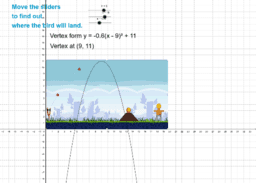### Angry Birds Love Parabolas

Activity

wolfteach

•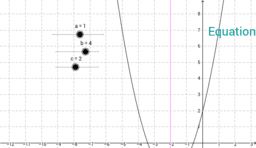### Move the axis of symmetry and the vertex - integers only

Activity

wolfteach

•### Quadratic equations and parabolas - 3 (complete vertex form)

Activity

wolfteach

•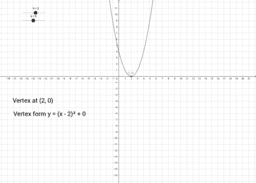### Quadratic equations and parabolas - 2 (Vertex form)

Activity

wolfteach

•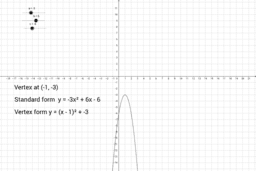### Quadratic equations and parabolas - 1

Activity

wolfteach

•### Moving a parabola using standard form of an equation.

Activity

wolfteach

•### Varying the vertex location of a parabola

Activity

wolfteach

•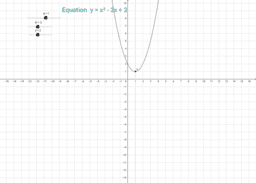Activity

wolfteach